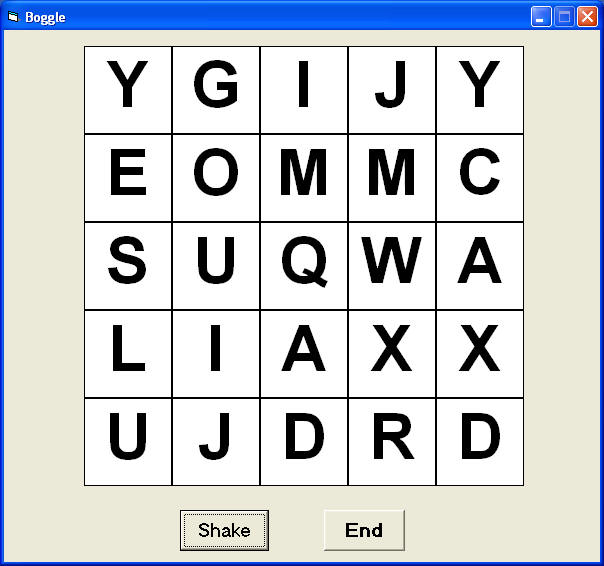# Boggle

Boggle is a type of words puzzle game where the players can form as many words as possible from the characters displayed on a nxn square. Words can be formed in many ways, from left to right, from right to left, top to bottom, bottom to top, diagonal, zigzag manner and etc.

In this program, we have designed a 5x5 boggle. Each time a player press the shake button, a different set of characters will appear. In order to do this, we use the randomize concept. This program generates an array of 26 characters and displays them on an array of 24 labels by using  a For...Next loop. The interface is as shown below:### The Code

Dim char(25) As String

Dim I As Integer
Dim J As Integer

Private Sub Command1_Click()
char(0) = "A"
char(1) = "B"
char(2) = "C"
char(3) = "D"
char(4) = "E"
char(5) = "E"
char(6) = "G"
char(7) = "H"
char(8) = "I"
char(9) = "J"
char(10) = "K"
char(11) = "L"
char(12) = "M"
char(13) = "N"
char(14) = "O"
char(15) = "P"
char(16) = "Q"
char(17) = "R"
char(18) = "S"
char(19) = "T"
char(20) = "U"
char(21) = "V"
char(22) = "W"
char(23) = "X"
char(24) = "Y"
char(25) = "Z"

Randomize Timer
For I = 0 To 24
J = Int((Rnd * 26))
Next

End Sub